English

MQL4 BookOperatorsOperator 'break'

# Operator 'break'

In some cases, for example, when programming some cycle operations, it could become necessary to break the execution of a cycle before its condition becomes false. In order to solve such problems, you should use the operator 'break'.

## Format of the Operator 'break'

The operator 'break' consists of only one word and ends in character ";" (semicolon).

`   break;                                         // Operator 'break'`

## Execution Rule of the Operator 'break'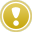The operator 'break' stops the execution of the nearest external operator of 'while', 'for' or 'switch' type. The execution of the operator 'break' consists in passing the control outside the compound operator of 'while', 'for' or 'switch' type to the nearest following operator. The operator 'break' can be used only for interruption of the execution of the operators listed above.

We can visualize the execution of the operator 'break' by the following example.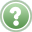Problem 14. Let's have a thread, 1 meter long. It is required to lay the thread in form of a rectangle with the maximum possible area. It is also required to find the area of this rectangle and the length of the sides with an accuracy to 1 mm by serial search in variations.

There can be an unlimited amount of rectangles of different sizes made of a unitary block of thread. Since the accuracy required by the problem statement makes 1 mm, we can only consider 499 variations. The first and the "thinnest" rectangle will have the dimensions of 1х499 mm, the second one will be 2х498 mm, etc., whereas the dimensions of the last rectangle will be 499х1 mm. We have to search in all these rectangles and find the one with the largest area.

As will readily be observed, there are repeated dimensions in the set of rectangles we are considering. For example, the first and the last rectangles have the same dimensions: 1х499 mm (the same as 499х1 mm). Similarly, the dimensions of the second rectangle will be the same as those of the last but one rectangle, etc. We have to make an algorithm that would search in all unique variations, whereas there is no need to search in the repeated ones.

First of all, let's estimate the relationship between the area and the length of the sides in a rectangle. As is easy to see, the first rectangle, with the dimensions of 1x499, has the smallest area. Then, with increase of the shorter side, the area of the rectangle will increase, too. As soon as they reach a certain value, the areas of the rectangles will start to decrease again. This relationship is shown in Fig. 44 below: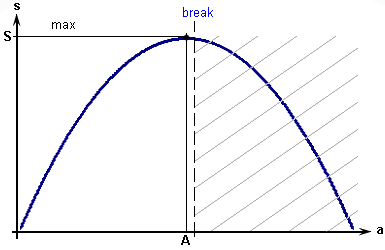Fig. 44.Relationship between the rectangle area and the length of one of its sides.

Looking at Fig. 44, we can easily come to the conclusion that we should find the maximum area by searching in variations starting from the first one, only as long as the area increases during calculations. As soon as it starts decreasing, we will break our search and exit the search cycle. Below is script rectangle.mq4 that implements such an algorithm.

`//--------------------------------------------------------------------// rectangle.mq4// The code should be used for educational purpose only.//--------------------------------------------------------------------int start()                         // Special function start()  {//--------------------------------------------------------------------   int   L=1000,                          // Specified thread length   A,                               // First side of the rectangle   B,                               // Second side of the rectangle   S,                               // Area of the rectangle   a,b,s;                           // Current values//--------------------------------------------------------------------   for(a=1; a<L/2; a++)             // Cycle operator header     {                              // Brace opening the cycle body      b=(L/2) - a;                  // Current value of the sides      s=a * b;                      // Current value of the area      if (s<=S)                     // Choose the larger value         break;                     // Exit the cycle      A=a;                          // Save the best value      B=b;                          // Save the best value      S=s;                          // Save the best value     }                              // Brace closing the cycle body//--------------------------------------------------------------------   Alert("The maximum area = ",S,"  A=",A,"  B=",B);// Message   return;                             // Function exiting operator  }//--------------------------------------------------------------------`

Let's watch how this program works. Variables are declared and commented on at the beginning of the program. The algorithm of problem solving itself is realized within the cycle 'for'. The initial value of the side a of the rectangle is specified as equal to 1 in Expression_1. According to the Condition, the values are searched in, as long as the size of the rectangle side a remains smaller than a half of the thread length. Expression_2 prescribes to increase the side length a of the current rectangle at each iteration step.

Variables a, b and s are the current variables, the values of which are searched in. Variables A, B and S are the search values. The second side b and the area s of the rectangle are calculated at the beginning of the cycle.

`      b = (L/2) - a;                   // Current values of the sides      s = a * b;                       // Current value of the area`

The condition of exiting the cycle is tested in the operator 'if':

`      if (s <= S )                     // Choose the larger value         break;                        // Exit the cycle`

If the newly calculated area s of the current rectangle turns out to be larger than the area S calculated at the preceding iteration, this new value of s becomes the best possible result. In this case, the Condition of the operator 'if' is not met, and the control is passed to the nearest operator that follows the operator 'if'. Below are the lines where we save the best results:

`      A = a;                           // Save the best value      B = b;                           // Save the best value      S = s;                           // Save the best value`

As soon as the program reaches the nearest closing brace, iteration is finished and the control is passed to the header of the operator 'for' to execute Expression_2 and test the Condition. If the length of the side a has not reached the specified limit as of the moment of testing, the cycle will continue to be executed.

The repeated cyclic calculations will continue until one of the following events takes place: either the length of the side a exceeds the predefined limits (according to the Condition of the operator 'for'), or the size of the calculated area s turns out to be smaller than a previously found value stored in variable S. There is a good reason to believe that the loop exit according to the condition of the operator 'if' will take place earlier:

`     if (s <= S )                     // Choose the larger value         break;                       // Exit the cycle`

Indeed, the cycle operator 'for' is composed in such a way that it searches in all possible variations without any exceptions (the half of the thread length, L/2, is the sum of two sides of the rectangle). At the same time, the maximum area of the rectangle will be reached somewhere in the middle of the searched set of variations. So, as soon as this happens (the area of the current rectangle s turns out to be less or equal to the previously reached value S), the control within the execution of the operator 'if' will be passed to the operator 'break' that, in its turn, will pass the control outside the operator 'for', to the following line:

`     Alert("The maximum area = ",S,"  A=",A,"  B=",B);// Message`

As a result of execution of the standard function Alert(), the following line will be printed:

 The maximum area = 62500 А=250 В=250

After that, the control will be passed to the operator 'return', which results in termination of the special function start(). This, in its turn, results in termination of the script and its being unloaded by the client terminal from the symbol window.

In this example, the operator 'break' stops (passes the control outside) the cycle operator 'for', namely, the cycle operator it is located in. Below is the block diagram of the cycle 'for' with a special exit: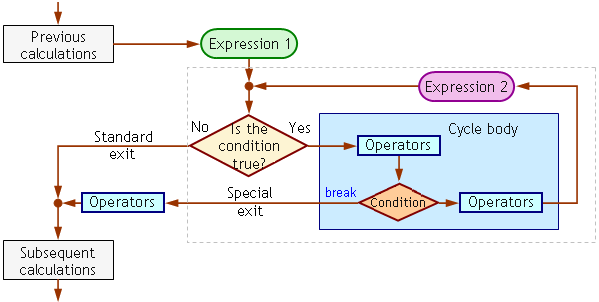Fig. 45. Block diagram of the cycle 'for' using the operator 'break' (rectangle.mq4).

As is seen from the diagram, there are two cycle exits: a normal exit (cycle exit resulting from triggering of the condition specified in the cycle operator header) and a special exit (the cycle body exit according to an additional condition and using the operator 'break').

It is difficult to overestimate the possibility to use a special exit in a cycle. In our example, the operator 'break' allows us to make an algorithm that performs only necessary calculations. An algorithm without a special exit would be inefficient: in this case, the repeated calculations would be performed, which would result in unreasoned waste of time and computational resources. The crosshatched region in Fig. 44 visualizes the region of parameters that were not processed in the above program (almost one half of all calculations!), which did not prevent us from obtaining the correct result.

The necessity to use effective algorithms becomes especially obvious, when the program execution time stretches to seconds and even minutes, which can exceed the time interval between ticks. In this case, there is a risk to omit processing of some informative ticks and, as a result, to lose control of your trading. Besides, you can feel the reduced time taken by calculations especially deeply if you test your programs in the Strategy Tester. Testing sophisticated programs on a long history can take hours and even days. The halved time taken by tests is a very important feature, in this case.

The Rule states that the operator 'break' stops the nearest external operator. Let's see how a program works that uses nested cycles.Problem 15. Using the algorithm of Problem 14, search the shortest thread multiple of 1 meter and sufficient to form a rectangle with an area of 1.5 m².

In this problem, we should linearly search in the available thread lengths and calculate the maximum area for each thread length. The solution of Problem 15 is realized in the script named area.mq4. The solution variations are searched in two cycles: internal and external. The external cycle searches in the thread lengths with the step of 1000 mm, whereas the internal one finds the maximum area for the current thread length. In this case, the operator 'break' is used to exit both the external and the internal cycle.

`//--------------------------------------------------------------------------// area.mq4// The code should be used for educational purpose only.//--------------------------------------------------------------------------int start()                             // Special function start()  {//--------------------------------------------------------------------------   int   L,                                  // Thread length   S_etalon=1500000,                   // Predefined area (m²)   S,                                  // Area of the rectangle   a,b,s;                              // Current side lengths and area//--------------------------------------------------------------------------   while(true)                         // External cycle for thread lengths     {                                 // Start of the external cycle      L=L+1000;                        // Current thread length of в mm      //--------------------------------------------------------------------      S=0;                             // Initial value..       // ..for each dimension      for(a=1; a<L/2; a++)             // Cycle operator header        {                              // НStart of the internal cycle         b=(L/2) - a;                  // Current side lengths         s=a * b;                      // Current area         if (s<=S)                     // Choose the larger value            break;                     // Exit internal cycle         S=s;                          // Store the best value        }                              // End of the internal cycle      //--------------------------------------------------------------------      if (S>=S_etalon)                 // Choose the larger value        {         Alert("The thread length must be ",L/1000," m.");// Message          break;                         // Exit the external cycle        }     }                                 // End of the external cycle//--------------------------------------------------------------------------   return;                              // Exit function operator  }//--------------------------------------------------------------------------`

The internal cycle works here like it did in the solution of the preceding problem. The operator 'break' is used to exit the cycle 'for', when the maximum area is found for the given thread length. It must be noted that the operator 'break' specified in the internal cycle 'for' passes the control to the operator that follows the brace closing the cycle 'for', and stops the cycle in this manner. This phenomenon does not influence the execution of the external cycle operator 'while' in any way.

As soon as the operator 'break' triggers in the internal cycle 'for', the control is given to the operator 'if':

`   if (S >= S_etalon)                              // Choose the larger value      {       Alert("The thread length must be ",L/1000," m.");// Message        break;                                      // Exit the external cycle      }`

In the operator 'if' we check whether the found area is more or equal to 1.5 m², the minimal value allowed by the problem statement. If yes, then the solution is found and there is no sense to continue calculating; the control will be passed to the body of the operator 'if'. The executable part of the operator 'if' is composed of only two operators, the first of which displays the message about the found solution on the screen:

 The thread length must be 5 m.

The second operator, 'break', is used to exit the external cycle 'while' and, subsequently, to exit the program. The block diagram of the algorithm realized in the program area.mq4 is shown below: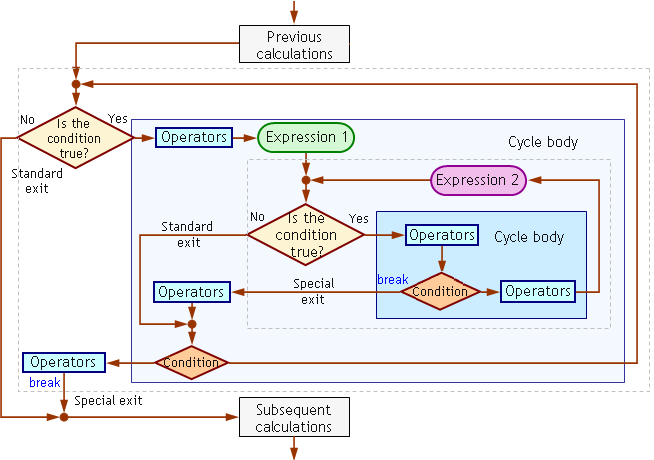Fig. 46. Block diagram of the program that realizes the possibility of special exit from internal and external cycles (area.mq4).

As is seen from the diagram, there are both a normal and a special exit for each cycle. Each operator 'break' stops its corresponding cycle, but it does not have any effect to the other cycle; each special exit correspond with its own operator 'break'. The same operator 'break' cannot be used in both cycles as special exit. In this case, each cycle has only one special exit. However, generally, it is possible to use several operators 'break' to make several special exits in one cycle.Please note that the Condition in the header of the external cycle 'while' is (true), i.e., a text that does not contain a variable, the value of which would change during the execution of the program. This means that the program will never exit the cycle 'while' under the Condition specified in the header. In this case, the only possibility to exit the cycle is to use the operator 'break'.

In this example, the algorithm is constructed in such a way that, in fact, the program uses only special exit to exit both the internal and the external cycle. However, you shouldn't think that the use of the operator 'break' always results in constructing algorithms that imply only special exits. In the algorithms of other programs, it is quite possible that the program reaches the condition and uses the normal exit to exit its cycles.

How to use the operator 'break' to pass the control outside the operator 'switch' is considered in the section Operator 'switch'.Cycle Operator 'for'Operator 'continue'